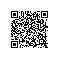# 写代码容易，编程并不容易“经验告诉我，我会颇具气势地拍、打和敲击键盘，但那并不是编程。”

1. class Stack
2.   # init stack
3.   def initialize
4.      = Array.new()
5.   end
6.   # put a new item at the end of the stack
7.   def push(x)
8.     .push x
9.   end
10.   # get the last item in the stack
11.   def grab
12.     .pop
13.   end
14.   # is the set empty true false bool
15.   def empty?
16.     .empty?
17.   end
18. end
19. # implemented stack
20. s = Stack.new
21. s.push 'a'
22. s.push 'b'
23. s.push 'c'
24. s.inspect #<Stack:0x48b66454 =["a""b""c"]>
25. puts s.grab # "c"
26. puts s.grab # "b"
27. puts s.grab # "a"
28. s.grab.inspect # nil

1. # ruby Queue Class
2. q = Queue.new
3. q << 'a'
4. q << 'b'
5. # Tests Examples using Queue
6. puts q.length  # prints 2
7. puts q.pop # prints a
8. puts q.length # prints 1
9. puts q.pop # prints b
10. puts q.length #prints 01. print " <h3> Display Weekdays: </h3> "
2. // Looping in a view ... should have been factored diff
3. foreach($imageRecords["display"as$ => $displayRecords) { 4. // WTF is this a nested foreach 5. foreach($displayRecords as $value =>$dispRecord){
6.        $tempWeekdayValuesArray = array(); 7. if($value === "weekdays" && !isnull($dispRecord)) { 8. // 3rd nested foreach WTF! 9. foreach($dispRecord as $weekday =>$weekbool) {
10.                  // :( condition foreach day ::SHAME::
11.                  if($weekday == "monday" &&$weekbool == 1) {
12.                     // logic removed
13.                   }
14.               }
15.
16.            }
17.       }使用钉钉扫一扫加入圈子
+ 订阅